# HBSE 10th Class Maths Notes Chapter 12 Areas Related to Circles

Haryana State Board HBSE 10th Class Maths Notes Chapter 12 Areas Related to Circles Notes.

## Haryana Board 10th Class Maths Notes Chapter 12 Areas Related to Circles

Introduction
In previous classes, we have learnt about methods of finding perimeters and areas of simple plane figures such as parallelogram, square, rectangle, rhombus and circle, In this chapter, we shall discuss review of the measurement of area and perimeter (circumference) of a circle, and leam about the concept of sector and a segment of a circle and their areas. We shall also learn about problems on finding the areas of some combinations of plane figures involving circles or parts of circles.

1. Circle: A circle is the locus of points which moves in such a way that its distance from a fixed point is constant.
2. Radius: A line segment joining the centre and a point on the circle is called its radius. The plural of radius is radii.
3. Diameter: The chord, which passes through the centre of the circle, is called a diameter of the circle.
4. Circumference: The length of the complete circle is called its circumference
OR
The perimeter of a circle is called its circumference.
5. Chord: A chord of a circle is a line segment joining any two points on the circle.
6. Arc: A piece of a circle between two points is called an are.
7. Sector of a circle: The region between an arc and the two radii, joining the centre to the endpoints of the arc is called a sector of the circle
8. Segment of the circle: The region between a chord and either of its arc is called a segment of the circular region or simply a segment of the circle.
9. Circular region: The region consisting of all points which are either on the circle or lies inside the circle is called the circular region or circular disc.
10. Semi-circular region: When two arcs are equal, that is, each is a semi-circle, then both segments and both sector become the same and each is known as the semi-circular region.
11. Concentric Circles: The circles which have same centre and different radii are called concentric circles.Perimeter and area of a circle – A Review
Let us recall that a circle is the set of all those points in a plane each of which is at constant distance from a fixed point in that plane.

The fixed point is called the centre and given constant distance is known as the radius of the circle. The distance covered by travelling once around a circle is its perimeter usually called its circumference.

We know that the ratio of circumference of a circle to its diameter is constant and this constant ratio is denoted by π (a Greek letter, read as pie).
$$\frac{\text { Circumference }}{\text { Diameter }}=\pi$$
⇒ π = $$\frac{\mathrm{C}}{2 r}$$ ⇒ C = 2πr
Where C is circumference and r is radius of circle.
Here π is an irrational number. For practical purposes, we generally take the value of π as $$\frac{22}{7}$$ or 3.14, approximately.

(i) Area of a circle
Area of a circle = πr2
where, r is the radius of the circle.
(ii) Perimeter and Area of Semi-circle
(a) Perimeter of semi-circle = $$\frac{1}{2}$$ × 2πr + 2r
(b) Area of semi-circle = $$\frac{1}{2}$$πr2
where r is the radius of a circle.

(iii) Perimeter and area of the quadrant of a circle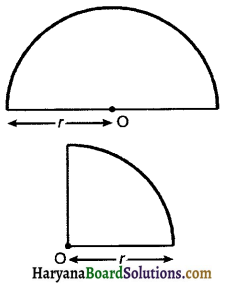If r is the radius of the circle
= $$\frac{1}{4}$$ × 2πr + 2r
= $$\frac{\pi r}{2}+2 r=r\left(\frac{\pi}{2}+2\right)$$
(b) Area of quadrant = $$\frac{1}{4}$$πr2

(iv) Area enclosed by two concentric circles
If R and r are radii of two concentric circles then
Area enclosed by them = πR2 – πr2 = π(R2 – r2)
= π(R + r)(R – r)
1. The distance covered by a rotating wheel in one revolution is equal to the circumference of the wheel.
2. The number of revolutions completed by a rotating wheel in one minute
$$=\frac{\text { distance covered in one minute }}{\text { circumference of wheel }}$$
3. When two circle touch internally distance between their centres = difference of their radii
4. When two circle touch externally, distance between their centres = sum of their radii.Areas of a sector and segement of a circle
(a) Sector of the circle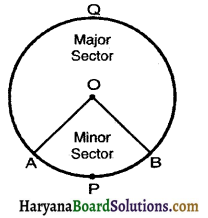The portion of circular region enclosed by two radii And corresponding arc is called a sector of the circle.
Let APB be in arc of a circle whose centre is O. Then region bounded by radii OA, OB and arc APB is called the sector of the circle. The sector OAPB is Major segment called the minor sector and OAQB is called the major sector. The angle of major sector is 360° – ∠AOB.

(b) Segment of a circle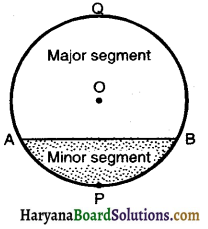The circular region enclosed between a chord and corresponding arc is called a segment of a circle.
Let AB be a chord of the circle with centre O. Then shaded region APB is a minor segment of the circle and AQB is a major segment of the circle.

Length of an arc and area of a sector of a circle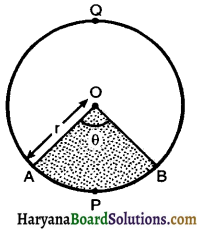1. Write the length of an arc of a sector of sector with radius and angle with degree measure θ.
Let OAPB be a sector of a circle with centre O and radius r such that ∠AOB = θ. If θ then AB is minor are of the circle.
If θ is increases then length of arc AB increases. When an are subtends an angle 180°, i.e., ∠AOB = 180°.
Then length of arc = Circumference of semicircle
= πr
If the arc subtends an angle of at the centre.
Then its arc length (l)
= $$\frac{\theta}{180}$$ × πr ……(1)
(l) = $$\frac{\theta}{360}$$ × 2πr
⇒ l = $$\frac{\theta}{360}$$ (Circumference of the circle)
When an arc subtends an angle 180°, at the centre the corresponding sector is semi-circular region of area = $$\frac{\pi r^2}{2}$$
If the arc subtends an angle at the centre then area of corresponding sector (A),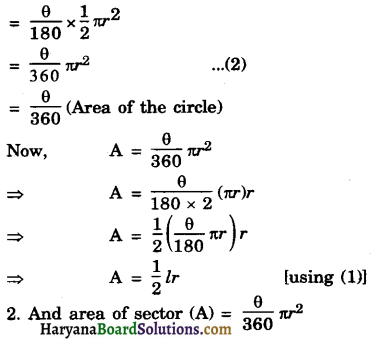Area of a segment of a circle
Let r be the radius of the circle with centre O. Let AB be a chord make a minor segment APB.
Let ∠AOB Draw OC ⊥ AB.
Area of minor segment APB = Area of sector OAPB – Area of ΔAOB
= $$\frac{\theta}{360^{\circ}} \pi r^2$$ – $$\frac{1}{2}$$AB × OC
= $$\frac{\theta \pi r^2}{360^{\circ}}-\frac{1}{2}$$ × 2AC × OC …… (1)
In right ΔACO, we have
$$\sin \frac{\theta}{2}=\frac{\mathrm{AC}}{\mathrm{OA}}$$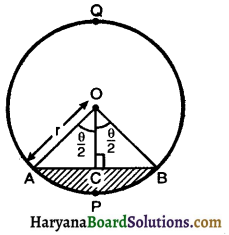AC = OA $$\frac{\theta}{2}$$sin = r sin$$\frac{\theta}{2}$$ and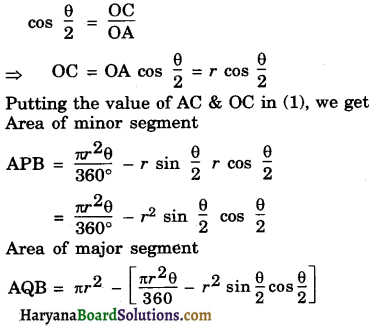Areas of combinations of plane figures
In this section we will learn to calculate the areas of some plane figures & designs which are combinations of more than one plane figures such that flowerbeds, drain covers, window design, designes on table covers etc. We illustrate the process of calculating areas of these figures through some examples.